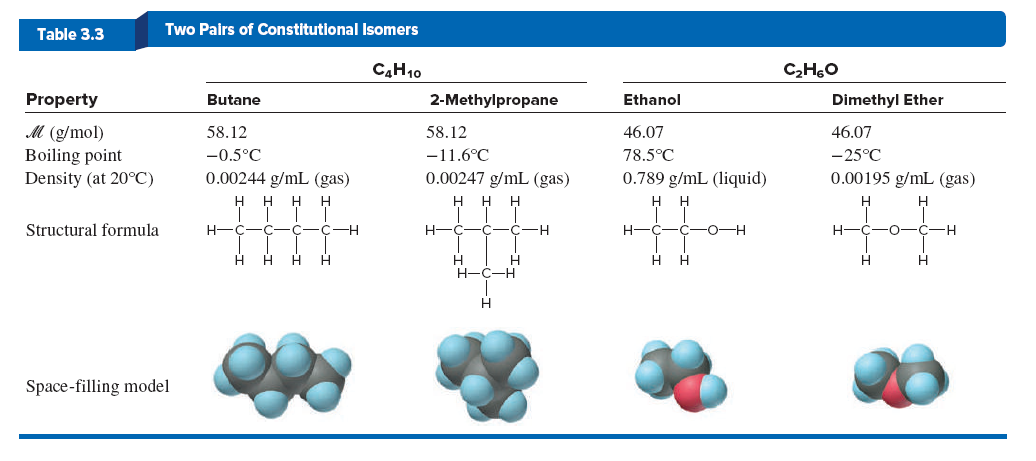# Problem: Dimethyl ether (CH 3OCH3) and ethanol (CH 3CH2OH) are constitutional isomers (see the following Table).(c) Calculate ΔH°rxn for the conversion of ethanol to dimethyl ether.

###### FREE Expert Solution
99% (129 ratings)
###### Problem Details

Dimethyl ether (CH 3OCH3) and ethanol (CH 3CH2OH) are constitutional isomers (see the following Table).

(c) Calculate ΔH°rxn for the conversion of ethanol to dimethyl ether.Frequently Asked Questions

What scientific concept do you need to know in order to solve this problem?

Our tutors have indicated that to solve this problem you will need to apply the Bond Energy concept. You can view video lessons to learn Bond Energy. Or if you need more Bond Energy practice, you can also practice Bond Energy practice problems.

What textbook is this problem found in?

Our data indicates that this problem or a close variation was asked in Chemistry: The Molecular Nature of Matter and Change - Silberberg 8th Edition. You can also practice Chemistry: The Molecular Nature of Matter and Change - Silberberg 8th Edition practice problems.